Related Articles

# NamedTuple in Julia

• Last Updated : 27 Feb, 2020

Tuples are a collection of heterogeneous and homogeneous datatypes written as an array, separated by commas. Tuples are basically immutable collections of distinct values. Tuples are different from arrays because arrays only take homogeneous datatypes as values.

NamedTuple is a type of Tuple whose elements can be called in the memory location not just by its index value, but also by a name of the value. Each value in a NamedTuple is represented by a unique value written as a symbol for that value.
Just like Tuples, NamedTuples are also immutable, which means neither the values nor their names once defined, can be changed.

Syntax:

`Tuple_name = (name1 = value, name2 = value2, ....)`
 `# Julia program to define NamedTuples``  ` `# Creating an empty tuple``tupl1 ``=` `()``println(isempty(tupl1))``  ` `# Creating a NamedTuple with similar values``tupl2 ``=` `(a ``=` `1``, b ``=` `2``, c ``=` `4``, d ``=` `5``)``println(tupl2)`` ` `# Creating a NamedTuple with mixed values``tupl3 ``=` `(a ``=` `1``, b ``=` `2``, c ``=` `"Hello Geeks"``)``println(tupl3)`

Output: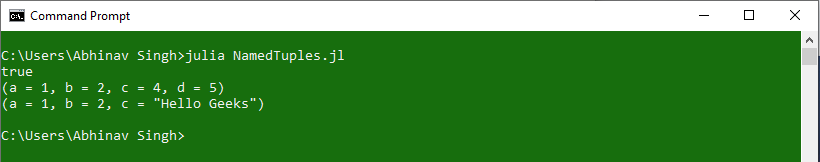#### Accessing elements from a NamedTuple

Elements from a named tuple can be accessed by using the field index assigned to them or by using the name assigned to the values.
Syntax:

```Tuple_Name[Index_value]
or
Tuple_Name[:Value_Name]
```

Example:

 `# Julia program to access elements ``# from a NamedTuple`` ` `# Creating a NamedTuple with similar values``tupl2 ``=` `(a ``=` `1``, b ``=` `2``, c ``=` `4``, d ``=` `5``)`` ` `# Accessing using Index value``println(tupl2[``2``])``println(tupl2[``4``])`` ` `# Creating a NamedTuple with mixed values``tupl3 ``=` `(a ``=` `1``, b ``=` `2``, c ``=` `"Hello Geeks"``)`` ` `# Accessing using assigned value name``println(tupl3[:b])``println(tupl3.c)`

Output: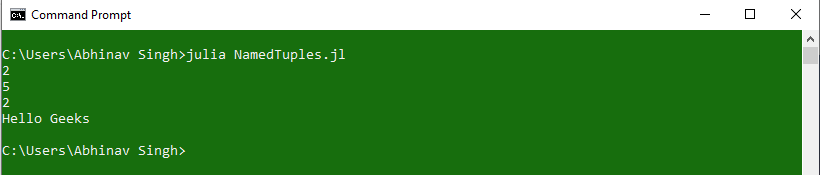Use of getindex() function:
Elements from a NamedTuple can also be accessed with the use of a predefined function in Julia, known as `getindex()`. This function accepts Tuple_Name and Key_value/Index_value as parameters and returns the element at that index.

Syntax:

```Element = getindex(Tuple_Name, Key_value)
Element = getindex(Tuple_Name, Index_value)
```

Example:

 `# Julia program to access keys and values ``# from a NamedTuple`` ` `# Creating a NamedTuple``tupl ``=` `(a ``=` `1``, b ``=` `2``, c ``=` `"Hello Geeks"``)`` ` `# Getting value using Key``Value1 ``=` `getindex(tupl, :b)`` ` `# Getting value using Index``Value2 ``=` `getindex(tupl, ``3``)`` ` `# Printing values``println(Value1)``println(Value2)`

Output: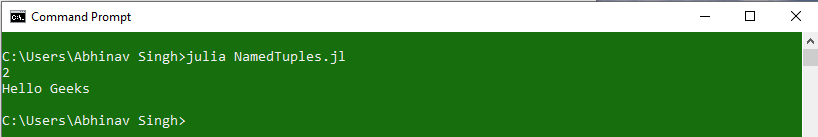## Accessing Keys and Values from a NamedTuple:

Keys are the names that are assigned to the values of a NamedTuple. Keys can be accessed in the form of a Tuple with the use of ‘keys’ keyword. Values, on the other hand, can be accessed with the use of ‘values’ keyword. These keywords generate a list of elements in the form of a Tuple.

Syntax:

```Keys = keys(Tuple_Name)
Values = values(Tuple_Name)
```

Example:

 `# Julia program to access keys and values ``# from a NamedTuple`` ` `# Creating a NamedTuple``tupl ``=` `(a ``=` `1``, b ``=` `2``, c ``=` `"Hello Geeks"``)`` ` `# Accessing keys``Keys ``=` `keys(tupl)`` ` `# Accessing Values``Values ``=` `values(tupl)`` ` `# Printing Keys and Values``println(Keys)``println(Values)`

Output: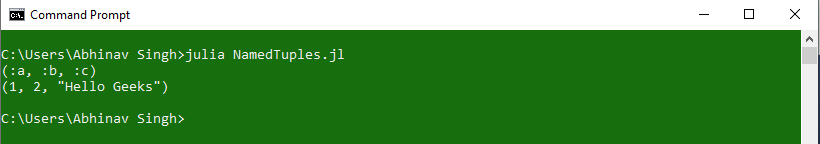#### Operations on NamedTuples

Julia allows to perform various operations on NamedTuples by using some pre-defined keywords. These operations are:

## Getting an array of Tuple Values using ‘`collect`‘:

Tuple values can also be represented as Arrays with the help of `collect` keyword. This keyword can also be used to print key-value pairs with the use of `pairs` keyword. This key value pairs is very useful for iterating over tuples.

Syntax:

```Array_Name = collect(Tuple_Name)
Array_Name = collect(pairs(Tuple_Name))```

Examples:

 `# Julia program to illustrate ``# the use of collect keyword`` ` `# Creating a NamedTuple``tupl ``=` `(a ``=` `1``, b ``=` `2``, c ``=` `3``, d ``=` `4``)`` ` `# Use of collect to get Array of values``Array ``=` `collect(tupl)`` ` `# Use of collect to get ``# Array of Key-value pair``Array2 ``=` `collect(pairs(tupl))`` ` `# Printing Arrays``println(Array)``println(Array2)`

Output: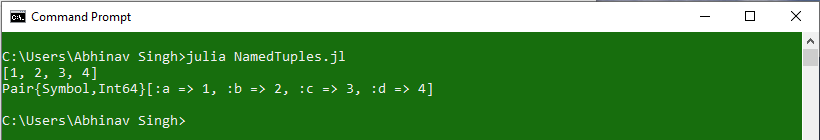## Use of ‘`Zip`‘ to create a Tuple:

A NamedTuple can also be created by defining Keys and Values separately as individual Tuples and then combining them together to form a NamedTuple. This can be done by using the keyword ‘zip‘. zip keyword is used along with a semicolon(:) placed inside the tuple.

Syntax:

```Keys = (Key1, Key2, Key3, ...)
Values = (Value1, Value2, Value3, ...)
Named_Tuple = (; zip(Keys, Values)
```

Example:

 `# Julia program to illustrate ``# the use of zip keyword`` ` `# Creating a tuple of Keys``Key_Tuple ``=` `(:a, :b, :c, :d)`` ` `# Creating a tuple of Values``Value_Tuple ``=` `(``1``, ``2``, ``3``, ``"Hello World"``)`` ` `# Zipping Keys and Values``Named_Tuple ``=` `(; ``zip``(Key_Tuple, Value_Tuple)...) `` ` `# Printing Values``println(``"Keys = "``, Key_Tuple)``println(``"Values = "``, Value_Tuple)``println(``"Tuple = "``, Named_Tuple)`

Output: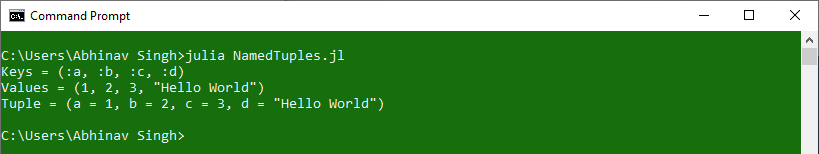## Use of ‘`merge`‘ to combine tuples:

Julia allows merging two or more NamedTuples into one tuple by using a keyword called ‘`merge`‘. When merging two tuples if both the tuples have a common key, then the value of the key in the tuple that is created most recently will be used in the final tuple.

Syntax:

`Final_tuple = merge(Tuple1, Tuple2, Tuple3, ...)`

Example 1:

 `# Julia program to illustrate ``# the use of merge keyword`` ` `# Creating first tuple``tupl1 ``=` `(a ``=` `1``, b ``=` `2``, c ``=` `3``)`` ` `# Creating second tuple``tupl2 ``=` `(d ``=` `4``, e ``=` `5``)`` ` `# Using merge operator``tupl3 ``=` `merge(tupl1, tupl2)`` ` `# Printing Final Tuple``println(tupl3)`

Output: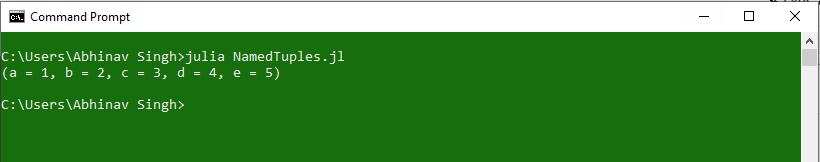Example 2:

 `# Julia program to illustrate ``# the use of merge keyword`` ` `# Creating first tuple``tupl1 ``=` `(a ``=` `1``, b ``=` `2``, c ``=` `3``)`` ` `# Creating second tuple``tupl2 ``=` `(b ``=` `"Hello"``, c ``=` `"Geeks"``)`` ` `# Using merge operator``tupl3 ``=` `merge(tupl1, tupl2)`` ` `# Printing Final Tuple``println(tupl3)`

Output: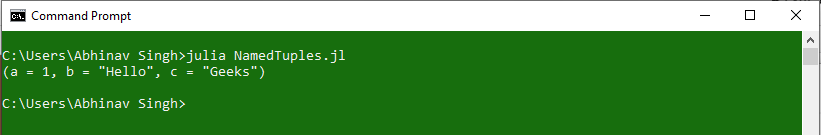My Personal Notes arrow_drop_up